# Solving similar triangles 1

##By Khan Academy

Sal solves two problems where a missing side length is found by proving that triangles are similar and using this to find the measure.# Special right triangles

##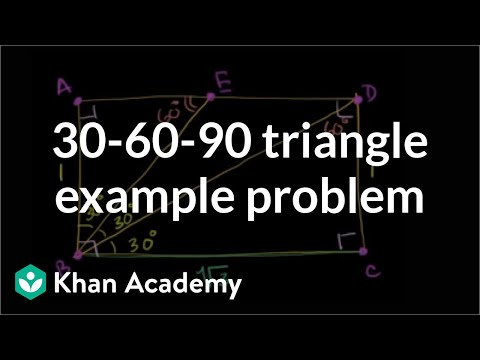By Khan Academy

Using what we know about 30-60-90 triangles to solve what at first seems to be a challenging problem# Special right triangles

##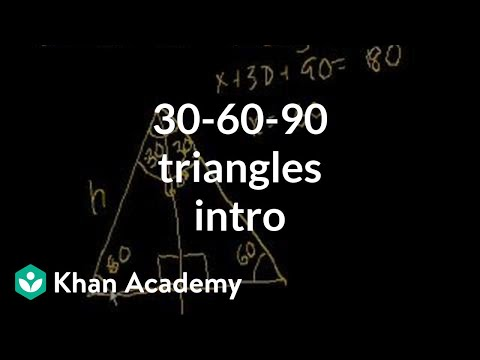By Khan Academy

A few more 45-45-90 examples and an introduction to 30-60-90 triangles.# Special right triangles

##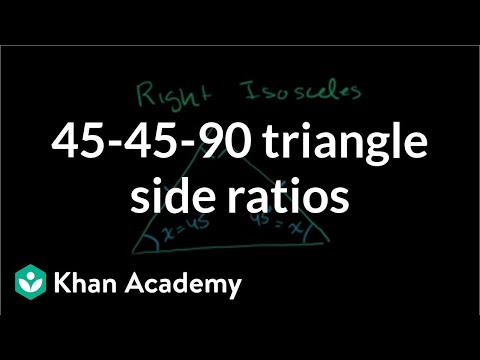By Khan Academy

Showing the ratios of the sides of a 45-45-90 triangle are 1:1:sqrt(2)# Similar triangles 1

##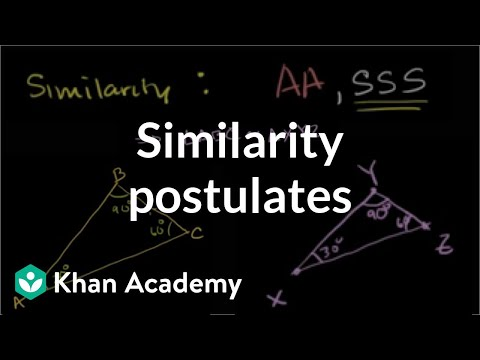By Khan Academy

Sal reviews all the different ways we can determine that two triangles are similar. This is similar to the congruence criteria, only for similarity!# Solving problems with similar and congruent triangles

##By Khan Academy

Sal uses the similarity of triangles and the congruence of others in this multi-step problem to find the area of a polygon.# Special right triangles

##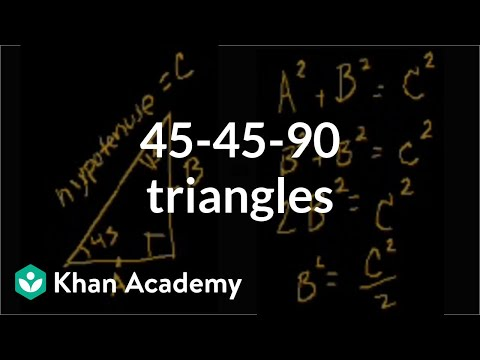By Khan Academy

Introduction to 45-45-90 Triangles# Find missing angles of triangles

##By Khan Academy

Three example problems involving isosceles and equilateral triangles (partly taken from Art of Problem Solving, by Richard Rusczyk).# Find missing angles of triangles

##By Khan Academy

Sal solves the following problem: The measures of two angles of an isosceles triangle are 3x+5 and x+16. Find all possible values of x.# Find missing angles of triangles

##By Khan Academy

Sal combines what we know about isosceles triangles and parallel lines with the power of algebra to solve the angles of an isosceles triangle.# Trigonometric functions of special angles

##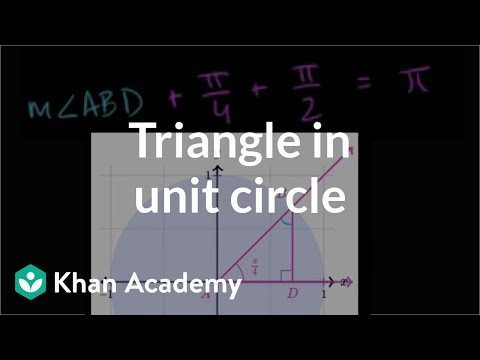By Khan Academy

Sal finds the trigonometric values ofï¿½ï¿½ï¿½ï¿½ï¿½ï¿½ï¿½ï¿½ï¿½ï¿½ï¿½ï¿½ï¿½ï¿½ï¿½ï¿½ï¿½ï¿½ï¿½ï¿½ï¿½ï¿½ï¿½ï¿½ï¿½ï¿½ï¿½/4.# Sine Values from Special Triangles

##By outofmylaboratory

YouTube presents Sine Values from Special Triangles an educational video resources on math.# Inscribed Angles

##By Wes Przybylski

This video provides a relatively quick lesson about inscribed angles and inscribed polygons. Uses diagrams to illustrate concepts.# Introduction to Geometry - 21 - Identifying Similar Triangles AA

##By thenewboston

"AA" stands for "Angle-Angle." This means that if two triangles are known to have two corresponding angles that are equal in measure, then the two angles are similar.# Using Corresponding Parts Of Congruent Triangles Are Congruent

##By MrPilarski

YouTube presents Using Corresponding Parts Of Congruent Triangles Are Congruent, an educational video resource on math.# Proving Triangles are Similar - YourTeacher.com - Geometry Help

##By yourteachermathhelp

Students learn the following theorems related to similar triangles. If an angle of one triangle is congruent to an angle of another triangle, and the lengths of the sides that include each angle are in proportion, then the triangles are similar (Side-Angle-Side Similarity Theorem, or SAS Similarity Theorem). If the lengths of the sides of two triangles are in proportion, then the triangles are similar (Side-Side-Side Similarity Theorem, or SSS Similarity Theorem). Students are then asked to determine whether given triangles are similar based on these theorems.# 9.7 Special Right Triangles (Lesson)

##By AutenMath

A review of practice problems using knowledge of special right triangles (30, 60, 90) and (45, 45, 90)# 9.7 Special Right Triangles (Practice)

##By AutenMath

9.7 Special Right Triangles (Practice)# Triangle Congruence by SSS and SAS - How to Prove Triangles Congurent

##By MrPilarski

YouTube presents Triangle Congruence by SSS and SAS - How to Prove Triangles Congurent, an educational video resource on math.# How to Solve Similar Triangles Applications: Flagpole Problem

##By Math Class with Terry V

YouTube presents How to Solve Similar Triangles Applications: Flagpole Problem, an educational video resource on math.